# Python库函数 Matplotlib安装及详解

Matplotlib 是一个Python的2D绘图库，它以各种硬拷贝格式和跨平台的交互式环境生成出版质量级别的图形。通过Matplotlib，开发者可以仅需要几行代码，便可以生成绘图，直方图，功率谱，条形图，错误图，散点图等。

1.PyCharm下安装Matplotlib：在PyCharm最下方找到Terminal，双击打开并输入：pip install Matplotlib，进行Matplotlib库函数的安装。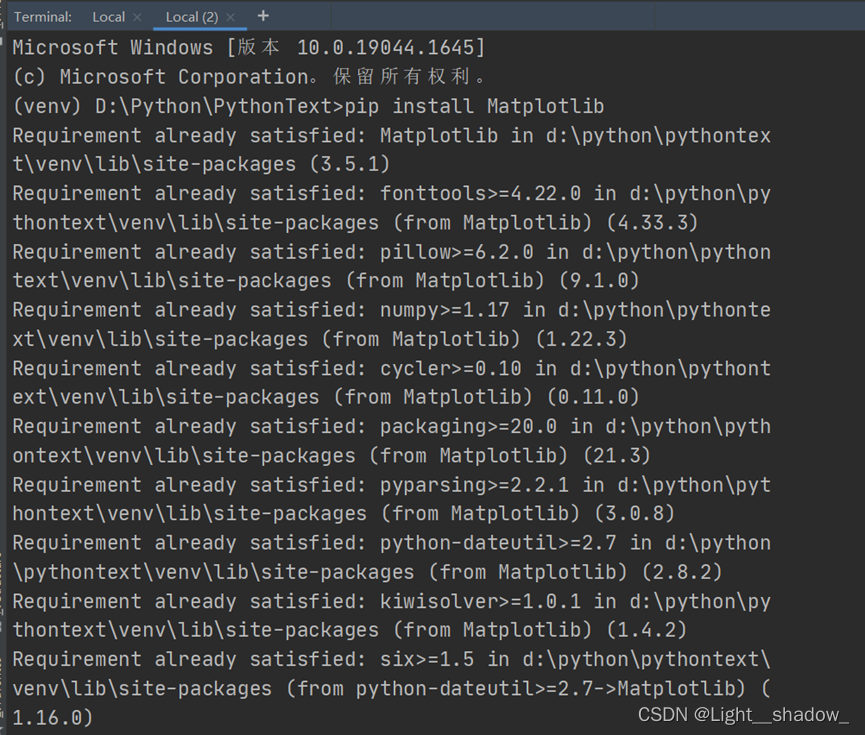2.Matplotlib 库函数安装完成，现在使用Matplotlib 库函数来绘制图形。

1. 柱状图
``````# 正常显示中文字体
plt.rcParams['font.sans-serif'] = ['Microsoft YaHei']
# 生成一张12*4的图
fig = plt.figure()
# 生成子图的位置
# 柱状图数据
x1 = [0.2, 1.7, 3, 4, 5]
y1 = [5, 20, 15, 25, 10]
# 子图绘图和标注的设置
ax1.bar(x1, y1)
# width=2
ax1.set(xlabel='横坐标', ylabel='纵坐标', title='柱状图')
#显示
plt.show()
``````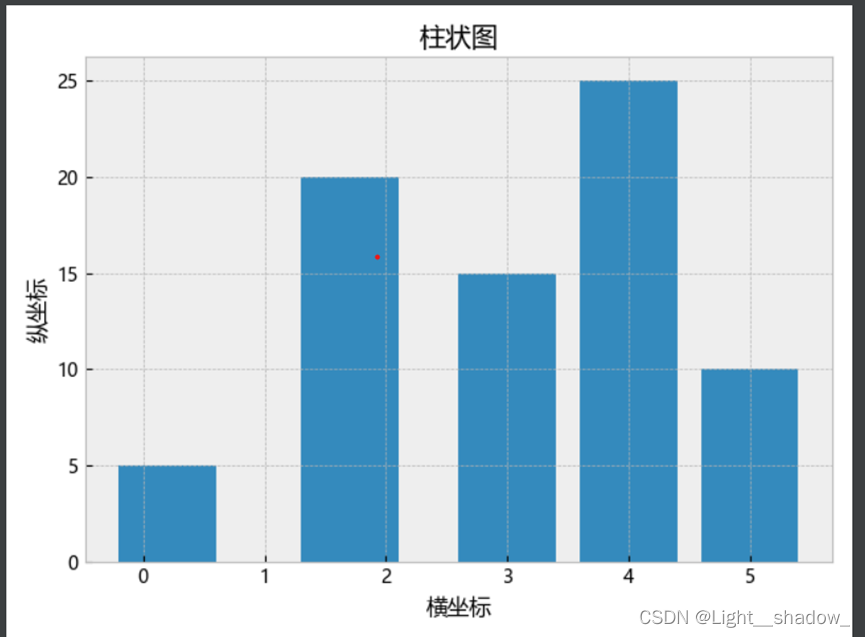1. plt.style.use('bmh') 它设置了柱形图的背景格式，除此之外还有许多其他格式，例如：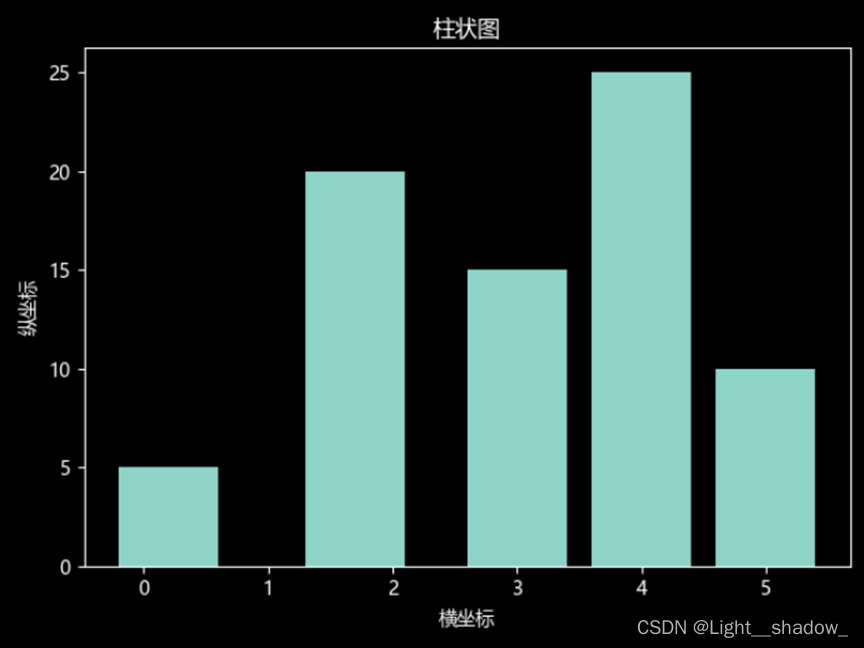``plt.style.use('dark_background')``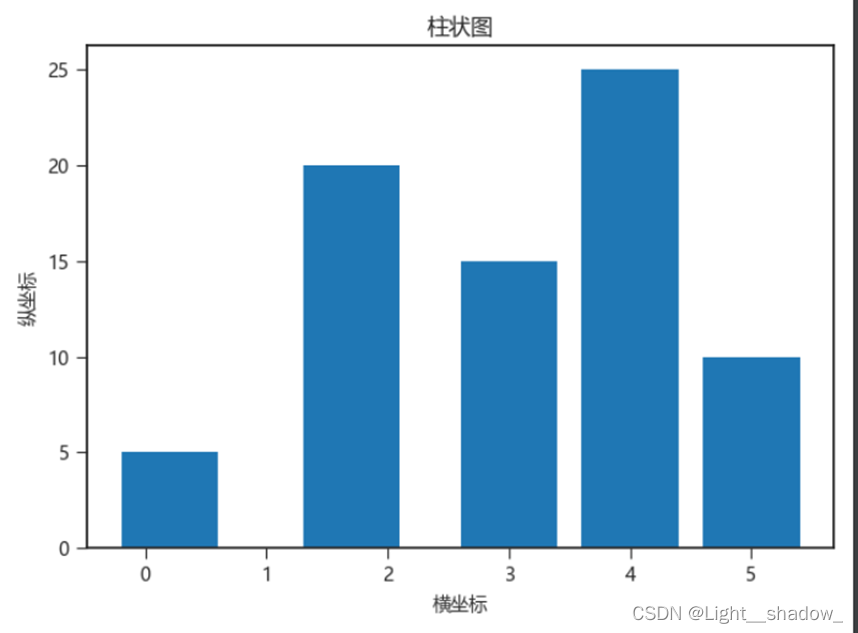``plt.style.use('seaborn-ticks')``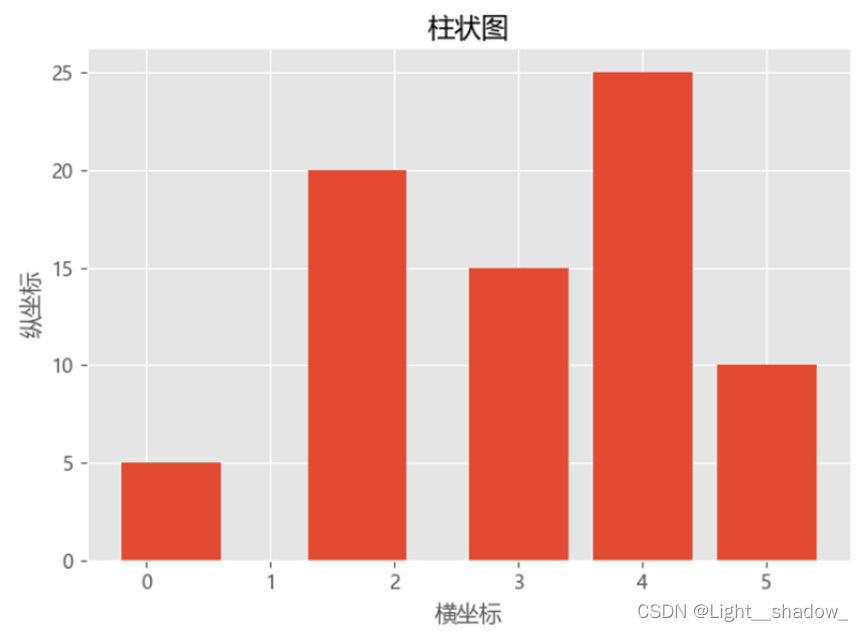``plt.style.use('ggplot')``
1. plt.rcParams['font.sans-serif'] ='SimHei':

#设置字体为SimHei显示中文；同时plt.rcParams还可以设置画图的分辨率，大小等信息。

,将生成的图片放大时，图片会变得模糊不清，``````plt.rcParams['savefig.dpi'] = 800 #图片像素
plt.rcParams['figure.dpi'] =800 #分辨率
``````

plt.rcParams不仅可以改变图片像素与分辨率还可以改变图片的大小：

``plt.rcParams['figure.figsize'] = (8.0, 4.0)``

Figure 1为未改变之前，Figure 2为设置plt.rcParams之后：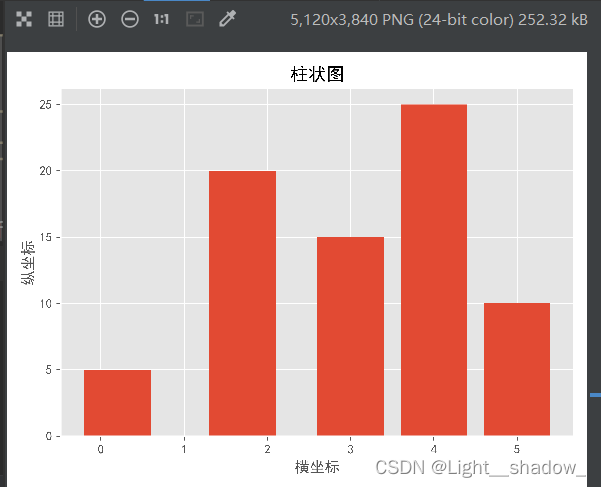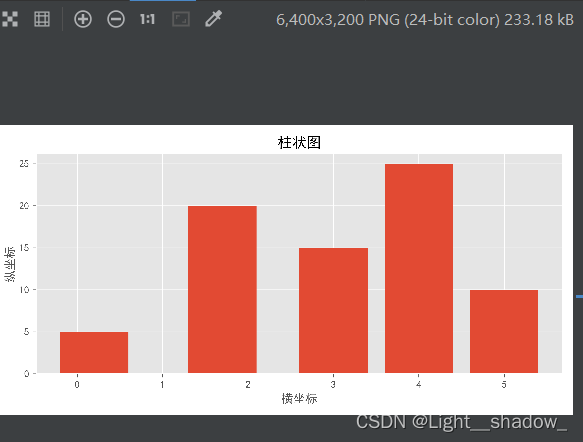plt.figure()：设置其中的参数，改变窗口的大小，背景颜色，边框颜色等：

``fig=plt.figure(figsize=(4,3),facecolor="blue")``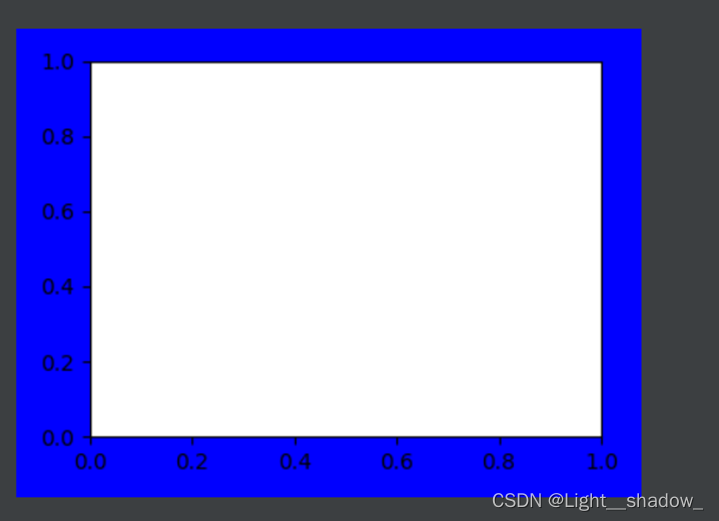plt.subplots()：如果只创建一个窗口，在subplots()中什么都不写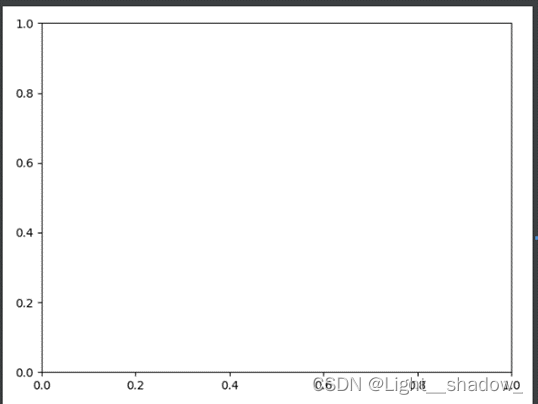``````import matplotlib.pyplot as plt
fig = plt.figure()
plt.show()
``````

如果要创建多个窗口，subplots(“行”，“列”，“该图片所在二维空间的位置”)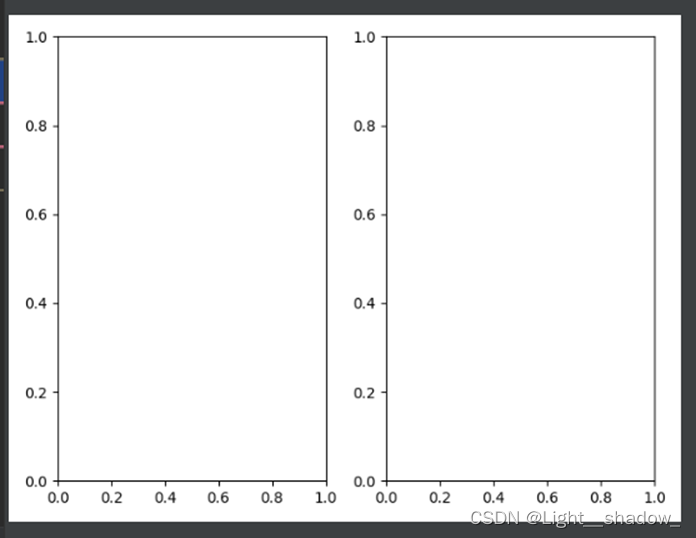``````ax = fig.add_subplot(121)
``````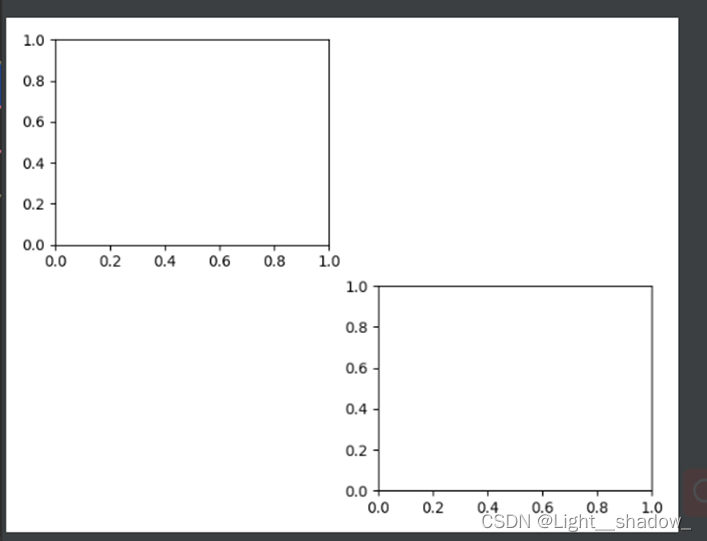``````ax = fig.add_subplot(221)
``````

``````# 柱状图数据
x1 = [0.2, 1.7, 3, 4, 5]
y1 = [5, 20, 15, 25, 10]
# 子图绘图和标注的设置
ax1.bar(x1, y1)
# width=2
ax1.set(xlabel='横坐标', ylabel='纵坐标', title='柱状图')
``````

``ax1.set(xlabel='横坐标', ylabel='纵坐标', title='柱状图')``

xlabel：设置横坐标的标注

ylabel：设置纵坐标的标注

title：设置图形标题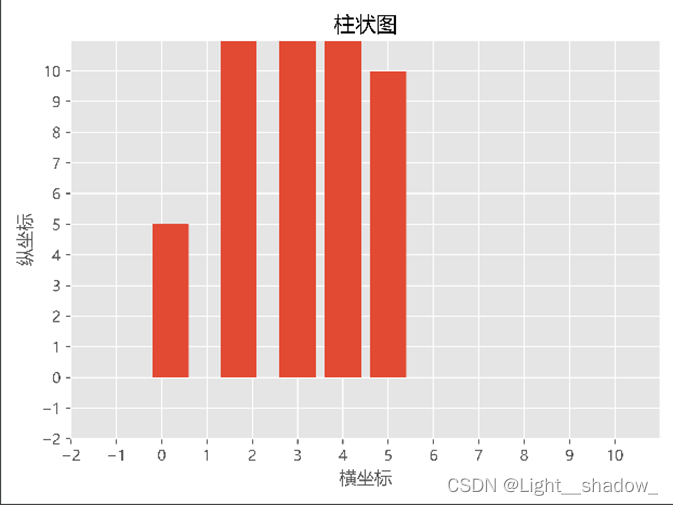``````ax1.set(xlim=(-5, 9), xticks=np.arange(-5, 9),#
xlim 设置X轴限制，xticks设置X轴刻度
ylim=(-5, 9), yticks=np.arange(-5, 9))
``````

ax1.bar(x1, y1)：根据x1和y1的参数绘制柱形图

bar:柱形图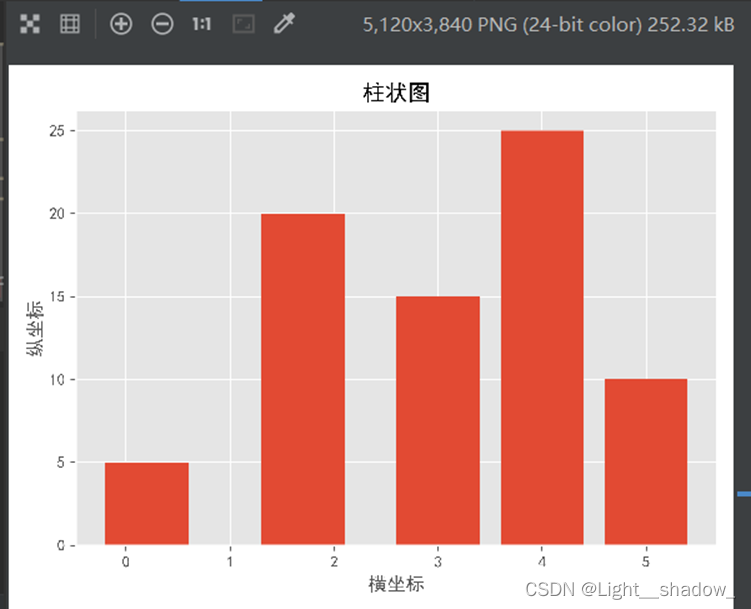ax1.plot(x1, y1)：根据x1和y1的参数绘制折线图

plot：折线图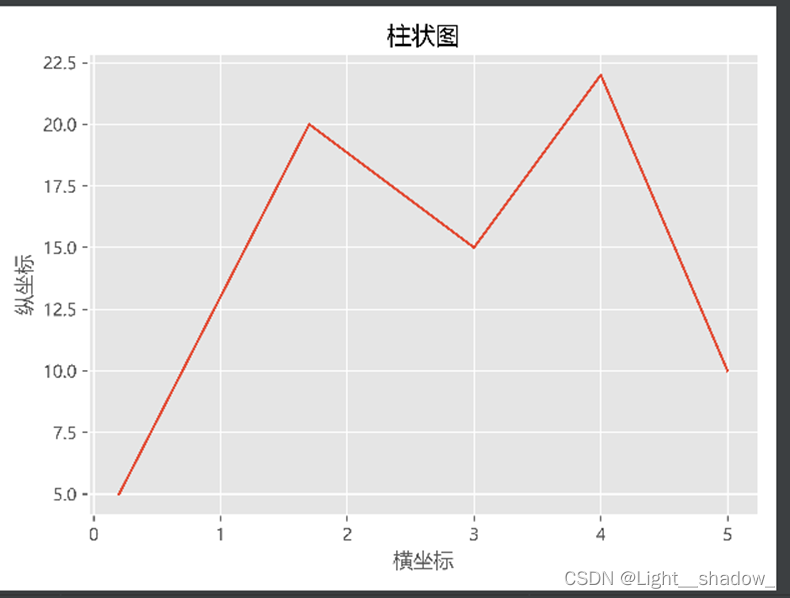ax1.scatter(x1, y1)：根据x1和y1的参数绘制散点图

scatter：散点图2.折线图

``````3)	#导入Matplotlib库函数
import matplotlib.pyplot as plt
import numpy as np
# 设置背景格式
plt.style.use('bmh')
# 正常显示中文字体
plt.rcParams['font.sans-serif'] =['Microsoft YaHei']
# plt.rcParams['figure.figsize'] = (8.0, 4.0)  # 设置figure_size尺寸
plt.rcParams['savefig.dpi'] = 800 #图片像素
plt.rcParams['figure.dpi'] =800 #分辨率
fig = plt.figure()
# 生成子图的位置
# 折线图数据
x1 = [0.2, 1.7, 3, 4, 5]
y1 = [5, 20, 15, 22, 10]
x2 = np.arange(0, 5)
y2 = [25, 2, 12, 30, 20]
ax1.plot(x1, y1, marker='*', ls='--', lw=3,ms=10 )
# 折线图 marker:标记形状；ms:标记的大小；ls：连接样式；
# lw：折线的粗细；c:折线的颜色
ax1.plot(x2,y2,marker='o',c='#992211', ms=10 )
ax1.set(xlabel='横坐标', ylabel='纵坐标', title='折线图')
plt.show()
``````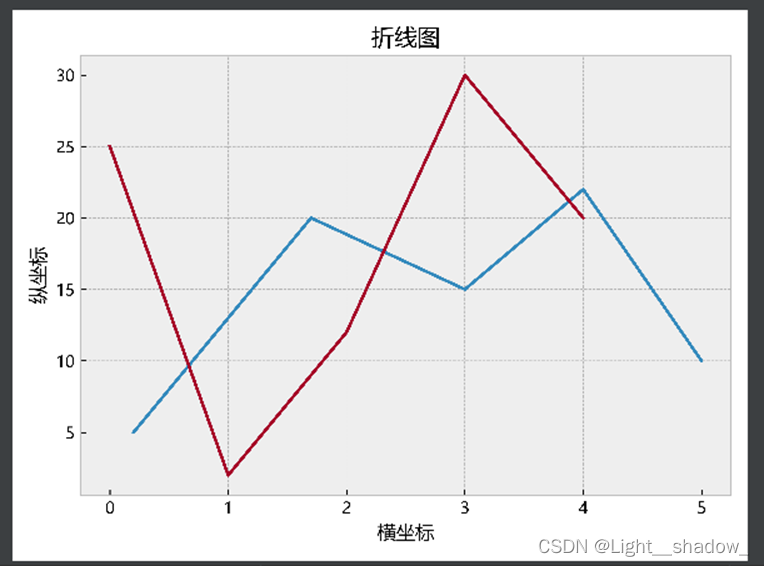1. 颜色

plot方法的关键字参数color(或c)用来设置线的颜色。可取值为：颜色名称或简写：

b: blue

g: green

r: red

c: cyan

m: magenta

y: yellow

k: black

w: white

2.样式

plot方法的关键字参数linestyle(或ls)用来设置线的样式。可取值为：

1. -, solid
2. –, dashed
3. -., dashdot
4. :, dotted
5. '', ' ', None

3.线条粗细

``````ax1.plot(x1, y1, marker='*', ls='--', lw=3,ms=10 )
# 折线图 marker:标记形状；ms:标记的大小；ls：连接样式；
# lw：折线的粗细；c:折线的颜色
ax1.plot(x2,y2,marker='o',c='#992211', ms=10 )
``````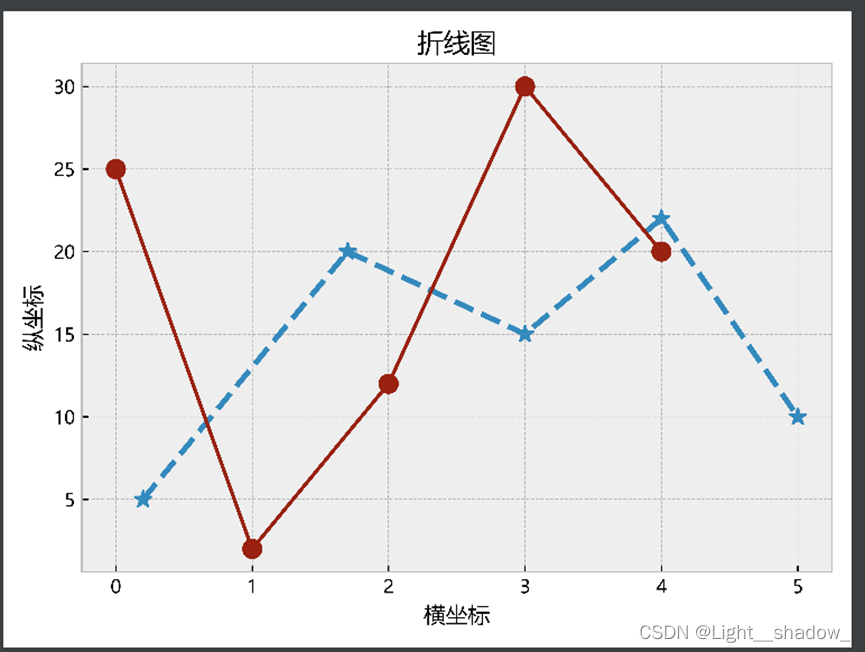3.直方图：又称质量分布图，是一种统计报告图，由一系列高度不等的纵条或线段表示数据分布情况。用横轴表示数据类型，纵轴表示分布情况。直方图是数值数据分布的精确图形表示。绘制连续性的数据，展示一组或多组数据的分布状况。

``````import numpy as np
import matplotlib.pyplot as plt
np.random.seed(19680801)
# example data
mu = 100  # mean of distribution
sigma = 15  # standard deviation of distribution
x = mu + sigma * np.random.randn(437)
num_bins = 50 #组距
fig, ax = plt.subplots()
plt.rcParams['font.sans-serif'] = ['Microsoft YaHei']
# 柱状图
# bins 将数据做 分箱 处理，然后统计每个箱内观察值的数量
# 选择出一个合适的区间（箱）宽度，并生成一个最能代表数据的直方图
# n, bins, patches = ax.hist(x, num_bins, density=True)
n, bins, patches = plt.hist(x, num_bins, facecolor='blue', alpha=0.5,density=True)
# 直方图函数，x为x轴的值，
# 绿色方块，色深参数0.5.返回n个概率，直方块左边线的x值，及各个方块对象
# 设置参数density=True实现数量的频率化,(趋势图)
y = ((1 / (np.sqrt(2 * np.pi) * sigma)) *
np.exp(-0.5 * (1 / sigma * (bins - mu))**2)) #组数=极差/组距
ax.plot(bins, y, '--')
ax.set_xlabel('横坐标')
ax.set_ylabel('纵坐标')
ax.set_title('直方图')
fig.tight_layout()
plt.show()
``````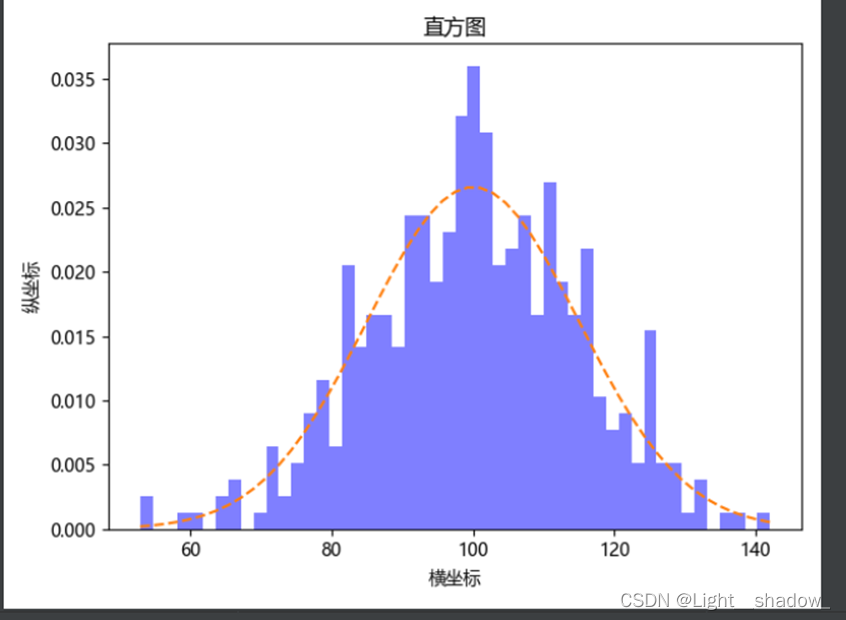改变bins组距，同时去掉density参数：

``````import numpy as np
import matplotlib.pyplot as plt
np.random.seed(19680801)
# example data
mu = 100  # mean of distribution
sigma = 15  # standard deviation of distribution
x = mu + sigma * np.random.randn(437)
num_bins = 10 #组距
fig, ax = plt.subplots()
plt.rcParams['font.sans-serif'] = ['Microsoft YaHei']
# 柱状图
# bins 将数据做 分箱 处理，然后统计每个箱内观察值的数量
y = ((1 / (np.sqrt(2 * np.pi) * sigma)) *
np.exp(-0.5 * (1 / sigma * (bins - mu))**2)) #组数=极差/组距
ax.plot(bins, y, '--')
ax.set_xlabel('横坐标')
ax.set_ylabel('纵坐标')
ax.set_title('直方图')
fig.tight_layout()
plt.show()``````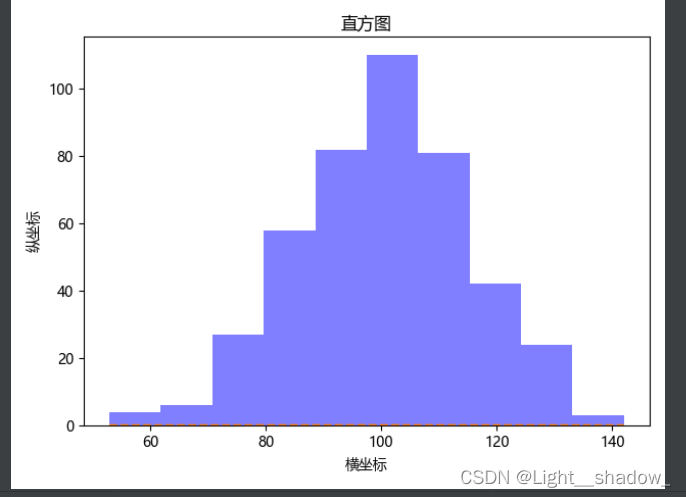hist的参数有：

hist(xbins=Nonerange=Nonedensity=Falseweights=Nonecumulative=Falsebottom=Nonehisttype='bar'align='mid'orientation='vertical'rwidth=Nonelog=Falsecolor=Nonelabel=Nonestacked=False*data=None**kwargs)

x（n，） 数组或 （n，） 数组序列

binsint 或 sequence 或 str，默认值：rcParams[“hist.bins”]（默认值：10

[1, 2, 3, 4]

rangetuple or None，默认值：无

Density布尔，默认值：False

Weights： weights（n，） 数组或无，默认值：无

counts, bins = np.histogram(data)

plt.hist(bins[:-1], bins, weights=counts)

cumulative布尔或 -1，默认值：False

Bottomarray-like, scalar, or Non，默认值：无

histtype{'bar'， 'barstacked'， 'step'， 'stepfilled'}， default： 'bar'

• “柱线”是传统的柱线型直方图。如果给出了多个数据，则条形将并排排列。
• “条形图”是一种条形图型直方图，其中多个数据堆叠在一起。
• “step”生成一个默认情况下未填充的行图。
• “步进填充”生成默认填充的行图。
• align{'left'， 'mid'， 'right'}， default： 'mid'

直方图条的水平对齐方式。

• “左”：条形图位于左侧素材箱边缘的中心。
• “中”：条形图位于条柱边缘之间居中。
• “右”：条形图位于右侧条柱边缘的中心。
• 方向{'垂直'， '水平'}默认值： '垂直'

如果为“水平”，则 barh 将用于条形直方图，底部 kwarg 将是左边缘。

rwidthfloat 或 None，默认值：无

条形的相对宽度作为条柱宽度的一小部分。如果 ，则自动计算宽度。None

如果 histtype 是“step”或“stepfilled”，则忽略。

logbool，默认值：False

如果 为True ，直方图轴将设置为对数刻度。

Color颜色或类似数组的颜色或无，默认值：无

颜色或颜色序列，每个数据集一个。缺省 （） 使用标准线颜色序列。None

labelstr 或 None，默认值：无

字符串或字符串序列以匹配多个数据集。条形图为每个数据集生成多个补丁，但只有第一个获得标签，因此该图例将按预期工作。

Stacked：布尔，默认值：False

如果 True，则多个数据堆叠在一起 如果 是False，则多个数据并排排列;如果 histtype 是 “step” ，则多个数据彼此堆叠在一起

binsarray

条柱的边缘。长度 nbins + 1（nbin 为最后一个条柱的左边缘和右边缘）。即使传入多个数据集，也始终为单个数组。

patchbarContainer 或单个多边形的列表或此类对象的列表

用于创建直方图的单个艺术家的容器或此类容器的列表（如果有多个输入数据集）。

官网文献：

matplotlib.axes.Axes.hist — Matplotlib 3.5.2 documentation